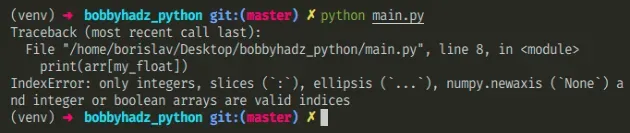# Only integers, slices (`:`), ellipsis (`...`), numpy.newaxis (`None`) and integer or boolean arrays are valid indicesSun Apr 24 20222 min read## Only integers, slices (`:`), ellipsis (`...`), numpy.newaxis (`None`) and integer or boolean arrays are valid indices#

The Python "IndexError: only integers, slices (`:`), ellipsis (`...`), numpy.newaxis (`None`) and integer or boolean arrays are valid indices" occurs when we use a non-supported type to index a numpy array. To solve the error, use the `int()` class to convert the value to an integer.Here is an example of how the error occurs.

main.py
```Copied!```import numpy as np

arr = np.array([1, 2, 3])

my_float = 1.0

# ⛔️ IndexError: only integers, slices (`:`), ellipsis (`...`), numpy.newaxis (`None`) and integer or boolean arrays are valid indices
print(arr[my_float])
``````

We used a float to index a numpy array which caused the error.

You can use the `int()` class to convert a floating-point number to an integer.

main.py
```Copied!```import numpy as np

arr = np.array([1, 2, 3])

my_float = 1.0

print(arr[int(my_float)])  # 👉️ 2
``````

The int class returns an integer object constructed from the provided number or string argument.

The constructor returns `0` if no arguments are given.

You might commonly get a float value if you use the division operator.

main.py
```Copied!```# 👇️ division
print(10 / 5)  # 👉️ 2.0

# 👇️ floor division
print(10 // 5)  # 👉️ 2
``````

Division `/` of integers yields a float, while floor division `//` of integers results in an integer.

The result of using the floor division operator is that of a mathematical division with the `floor()` function applied to the result.

If you aren't sure what type a variable stores, use the built-in `type()` class.

main.py
```Copied!```my_float = 1.0

print(type(my_float))  # 👉️ <class 'float'>
``````

The type class returns the type of an object.

You can also use slices or integer arrays to index a numpy array.

main.py
```Copied!```arr = np.array([[1, 2], [3, 4], [5, 6]])

print(arr[0: 2])  # 👉️ [[1 2] [3 4]]

print(arr[:, 0])  # 👉️ [1 3 5]

print(arr[0:2, 0])  # 👉️ [1 3]
``````

The first example selects the first 2 elements in the numpy array.

Indexes are zero-based and the `start` index is inclusive, whereas the `stop` index is exclusive.

The second example selects the fist element in each nested array.

The third example selects the first element in the first two nested arrays.

Use the search field on my Home Page to filter through my more than 1,000 articles.